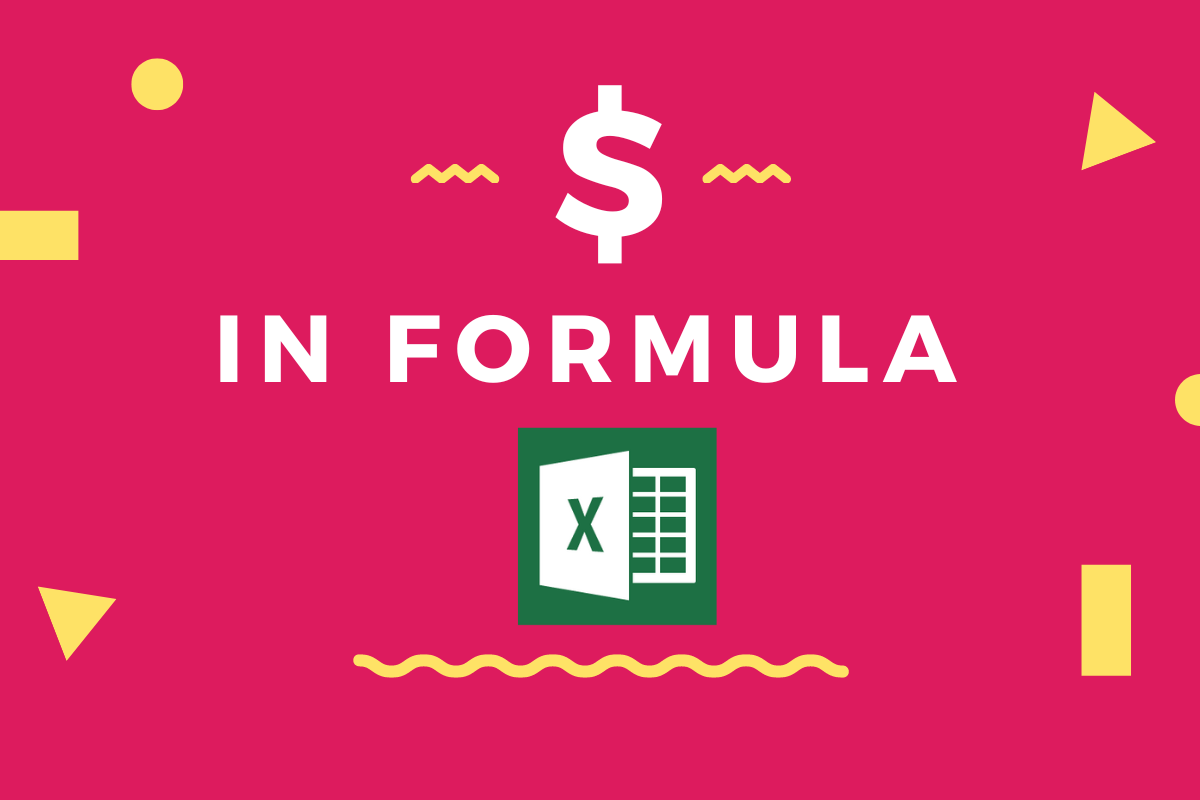# \$ in Formula in MS Excel – What Does it Mean? & Examples of UsageThe important selling point of MS Excel back in those days was its ease in using formulae and it stays that way to this very day. We can refer to the data in any cells & build umpteen formulae in MS Excel in such a way that the results of the formula get updated the moment one changes the values in those cells given as references.

But one needs to exercise special care when needed to copy or transfer the formula constructed in one cell to the others. We are going to explore just that in this article & following is the dataset which would be used throughout this article to demonstrate the use case of the ‘\$’ symbol within the formulae.

## What is \$ in formulas in excel?

The dollar (\$) sign is used to make the reference cells in an Excel formula sticky. Even if a formula cell is copied, the reference cells remain the same.

For example, if you write a formula =SUM(A2:A5) and then copy the cell to the next column, the referenced cells “A2:A5” will change to B2:B5. With the \$ sign, your formula because =SUM(\$A\$2:\$A\$5) and now, you can copy this formula to any column or cell on your sheet and it will continue to give you the sum of values in A2:A5 cells.

Let’s discuss this further.

The process of referring to cells within a formula is called ‘Referencing’ & there are 3 types of putting it into use.

• Method 1 – Normal Referencing
• Method 2 – Relative Referencing
• Method 3 – Absolute Referencing

## Method 1 – Normal Referencing:

Let us try to understand this by constructing a formula to find the buying price per unit for chips in the above example.

The total price of chips bought is given in cell N3 & the total quantity of chips bought is stated in cell M3. So, to find the unit price we have divided the Total price of chips by the Total quantity of chips.

Great! Logic has been applied & the formula is constructed. Can we copy this formula in P3 to the cells P4 & P5 as well for calculating the unit buying price of other items?

We might as well do that to see if it works!

The formula copied, when pasted to the other cells seems to have the correct references for the numerator & the denominator. This can be explained by the inherent feature of MS Excel to auto-adjust the references in the formula when copied in any direction, which in this case is downwards. Both the numerator & denominator are auto-adjusted one cell in the downward direction for each cell the formula is being pasted.

This approach of transferring the formula without the usage of ‘\$’ is called Normal Referencing.

## Method 2 – Relative Referencing:

Great! Now let’s copy the formula from the above cells & try pasting them in the adjacent column to find the unit selling price for each item.

Here’s what we get.

At the very first glance, one might be able to figure out that the numerator has been referenced to the correct cell, but the denominator instead of referring to the cell in ‘Qty’ column, is rather referenced to the cell in ‘Buying Price’ column.

The very feature of MS Excel which auto adjusts the references is to be blamed in this case, since copying the formula from column P to Q not only moves the cell reference of the numerator one cell to the right but also that of the denominator too!

How to tackle this tricky situation, you ask? With a simple ‘\$’ inclusion in the denominator. What the ‘\$’ sign does in a formula is to fixate the cell reference either to the row or the column before which it is placed upon. For instance,

\$A1 – All cells to which the formula is pasted will be referring to column A

A\$1 – All cells to which the formula is pasted will be referring to row 1

This technique of fixating either the row or the column & NOT BOTH while referencing is called Relative Referencing. So, let’s include a ‘\$’ before column M in the formula.

When the above formula is copied & pasted to the other cells, let’s see what happens!

It works fine! That’s what happens.

## Method 3 – Absolute Referencing:

What if there’s only one cell with a quantity like the one shown below?